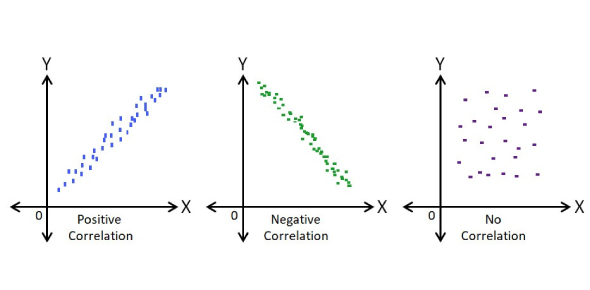# Scatter Plot And Correlation Exam! Quiz

11 Questions | Attempts: 1383SettingsThere are different ways in which people use to display data for presentation purposes. Using a scatter plot is the best method when it comes to showing the correlation between different subjects. This quiz is for students in my Intermediate Algebra class to take after they've read the website. Give it a try and get to see just how much you know.

• 1.
Correlation is a relationship between two sets of data.
• A.

True

• B.

False

• 2.
When making a scatterplot you want to always connect the dots (points)
• A.

True

• B.

False

• 3.
When you have collected your data you may find that certain x values may have more than one y-value, such as (7,90) and (7,85) and (7,100). This is not okay. You should get rid of (or ignore) all but one of these points.
• A.

True

• B.

False

• 4.
If the slope of a line drawn through the scatterplot is positive (rising from left to right), that means there is a positive correlation between the data.
• A.

True

• B.

False

• 5.
Not all data sets will have a positive correlation. It all depends on the data being examined.
• A.

True

• B.

False

• 6.
If the slope of a line drawn through the scatterplot is negative (falling from left to right), that means there is no correlation between the data.
• A.

True

• B.

False

• 7.
If the plot on the graph is scattered in such a way that it does not approximate a line (it does not appear to rise or fall), there is no correlation between the sets of data.
• A.

True

• B.

False

• 8.
What kind of correlation is shown in the following graph?
• A.

High Positive Correlation

• B.

Low Positive Correlation

• C.

High Negative Correlation

• D.

Low Negative Correlation

• E.

No Correlation

• 9.
What kind of correlation is shown in the following graph?
• A.

High Positive Correlation

• B.

Low Positive Correlation

• C.

High Negative Correlation

• D.

Low Negative Correlation

• E.

No Correlation

• 10.
Just because there is a strong correlation between data, does not necessarily mean that one set of data is causing the effect that is occurring in the other set of data.
• A.

True

• B.

False

• 11.
In the last example on the page, the scatterplot shows that the number of jars of strawberry jam sold in New York was causing an increase in the number of classical music CDs sold in Florida.
• A.

True

• B.

False

## Related TopicsBack to top
×

Wait!
Here's an interesting quiz for you.